#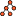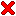Computing

Together with colleagues at ETH Zürich/Switzerland, we develop libraries for linear algebra, motion planning, and sensor data processing. The programming language is Java 8; packaging is with maven.

Our open-source software is specifically designed, manufactured and intended for use in the planning, construction, maintenance, control, and direct operation of nuclear facilities; aircraft navigation, control and communication systems; weapons systems; and direct life support systems.

Computing provides approximate strategies to real world problems and games.

It means that I, like God, do not play with dice
and do not believe in coincidence.
V in Vendetta

##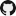Tensor - exact precision, multi-dimensional linear algebra

•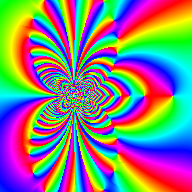Gamma function
•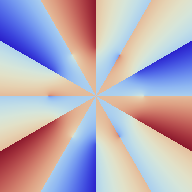Trigonometry
•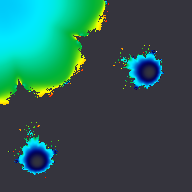Nylander's formula
•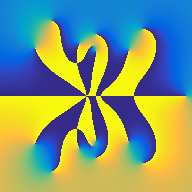Newton's method

The tensor library was developed with the following objectives in mind

• support for exact precision using integer fractions
• support for calculation with physical units
• suitable for use in safety-critical real-time systems
• API inspired by Mathematica

Integer fractions are suitable for use as keys in a hash table due to their unique encoding. Quantities with units are useful to assert the correctness of formulas in the physical domain. The tensor library is used for the operation of heavy and fast mobile robots.

The source code is hosted on github

 Tensor (Java 8, maven project) *idsc-frazzoli/tensor link
* Credits: The arithmetic for the scalar type Quantity was developed in collaboration with Micha Güdel from the Swisstrolley+ project. Joel Gächter contributed functionality to the probability distribution and the image processing packages.

The example below demonstrates the calculation of the Cholesky decomposition and the determinant of a matrix in exact precision. The entries of the matrix are integers with mixed physical units.

```Tensor matrix = Tensors.fromString( //
CholeskyDecomposition cd = CholeskyDecomposition.of(matrix);
System.out.println(cd.diagonal());
System.out.println(Pretty.of(cd.getL()));
```

gives

```{60[m^2], 5[rad^2], 1/3[kg^2]}
[
[             1              0              0 ]
[ 1/2[m^-1*rad]              1              0 ]
[  1/3[kg*m^-1]   1[kg*rad^-1]              1 ]
]
```
You could verify that this matrix is correct,
or you could go outside and play in the sunshine.
Keenan Crane

##Subare - Reinforcement Learning Library

•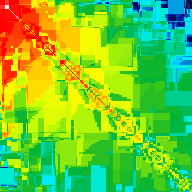Optimists

Java 8 implementation of algorithms, examples, and exercises from the 2nd edition (2016 draft) of Sutton and Barto: Reinforcement Learning. Our implementation was designed based on the objectives:

• the algorithms are implemented separate from the problem scenarios
• the math is in exact precision which reproduces symmetries in the results in case the problem features symmetries

The source code is hosted on github

 Subare (Java 8, maven project)idsc-frazzoli/subare link

List of algorithms:

• Iterative Policy Evaluation (parallel, from p.81)
• Value Iteration to determine V*(s) (parallel, from p.90)
• Action-Value Iteration to determine Q*(s,a) (parallel)
• First Visit Policy Evaluation (from p.100)
• Monte Carlo Exploring Starts (from p.107)
• Contant-alpha Monte Carlo (from p.127)
• Tabular Temporal Difference (from p.128)
• Sarsa: An on-policy TD control algorithm (from p.138)
• Q-learning: An off-policy TD control algorithm (from p.140)
• Expected Sarsa (from p.142)
• Double Sarsa, Double Expected Sarsa, Double Q-Learning (from p.145)
• n-step Temporal Difference for estimating V(s) (from p.154)
• n-step Sarsa, n-step Expected Sarsa, n-step Q-Learning (from p.157)
• Random-sample one-step tabular Q-planning (parallel, from p.169)
• Tabular Dyna-Q (from p.172)
• Prioritized Sweeping (from p.178)
For every complex problem there is an answer that is clear, simple, and wrong.
Henry Louis Mencken